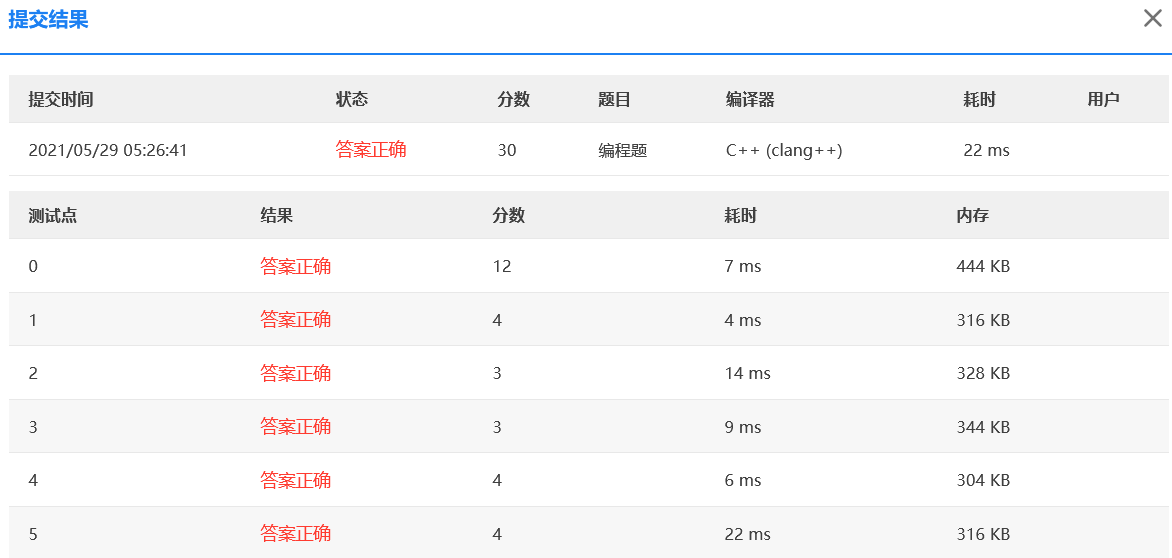﻿• 价格透明
• 信息保密
• 进度掌控
• 售后无忧# 【PAT甲级】1014 Waiting in Line (30 分)

Suppose a bank has N N windows open for service. There is a yellow line in front of the windows which devides the waiting area into two parts. The rules for the customers to wait in line are:

• The space inside the yellow line in front of each window is enough to contain a line with M M customers. Hence when all the N N lines are full, all the customers after (and including) the ( N M + 1 ) (NM+1) st one will have to wait in a line behind the yellow line.
• Each customer will choose the shortest line to wait in when crossing the yellow line. If there are two or more lines with the same length, the customer will always choose the window with the smallest number.
• C u s t o m e r i Customer_i ​​ will take T i T_i ​​ minutes to have his/her transaction processed.
• The first N N customers are assumed to be served at 8:00am.

Now given the processing time of each customer, you are supposed to tell the exact time at which a customer has his/her business done.

For example, suppose that a bank has 2 windows and each window may have 2 customers waiting inside the yellow line. There are 5 customers waiting with transactions taking 1, 2, 6, 4 and 3 minutes, respectively. At 08:00 in the morning, c u s t o m e r 1 customer_1 ​​ is served at w i n d o w 1 window_1 ​​ while c u s t o m e r 2 customer_2 ​​ is served at w i n d o w 2 window_2 ​​. C u s t o m e r 3 Customer_3 ​​ will wait in front of w i n d o w 1 window_1 ​​ and c u s t o m e r 4 customer_4 ​​ will wait in front of w i n d o w 2 window_2 ​​. C u s t o m e r 5 Customer_5 ​​ will wait behind the yellow line.

At 08:01, c u s t o m e r ​ 1 customer​_1 ​​ is done and c u s t o m e r ​ 5 customer​_5 ​​ enters the line in front of w i n d o w 1 window_1 ​​ since that line seems shorter now. C u s t o m e r 2 Customer_2 ​​ will leave at 08:02, c u s t o m e r 4 customer_4 ​​ at 08:06, c u s t o m e r 3 customer_3 ​​ at 08:07, and finally c u s t o m e r 5 customer_5 ​​ at 08:10.

## Input Specification:

Each input file contains one test case. Each case starts with a line containing 4 positive integers: N N ( ≤ 20 ≤20 , number of windows), M M ( ≤ 10 ≤10 , the maximum capacity of each line inside the yellow line), K K ( ≤ 1000 ≤1000 , number of customers), and Q Q ( ≤ 1000 ≤1000 , number of customer queries).

The next line contains K K positive integers, which are the processing time of the K K customers.

The last line contains Q Q positive integers, which represent the customers who are asking about the time they can have their transactions done. The customers are numbered from 1 to K K .

## Output Specification:

For each of the Q Q customers, print in one line the time at which his/her transaction is finished, in the format HH:MM where HH is in [08, 17] and MM is in [00, 59] . Note that since the bank is closed everyday after 17:00, for those customers who cannot be served before 17:00, you must output Sorry instead.
Sample Input:

2 2 7 5
1 2 6 4 3 534 2
3 4 5 6 7


Sample Output:

08:07
08:06
08:10
17:00
Sorry


### 解法 队列+模拟

• 顾客如何找到人数最少且序号最小的窗口；
• 如果顾客在17:00之前没有被服务到，他就不会被服务了，查询时输出 Sorry
• 如果顾客在17:00之前开始被服务，即使过了17:00的银行关门时间，也应该继续服务下去

#include <bits/stdc++.h>
using namespace std;
const int MAXC = 1010, BEGIN = 8 * 60, END = 17 * 60;
queue<int> wins;
int n, m, k, q, t, proc[MAXC], start[MAXC], last[MAXC], now = BEGIN, cur = 0;
int main() {
scanf("%d%d%d%d", &n, &m, &k, &q); //窗口,容量,顾客,查询
for (int i = 0; i < k; ++i) scanf("%d", &proc[i]);
for (int i = 0; i < m && cur < k; ++i) {
for (int j = 0; j < n && cur < k; ++j) {
start[cur] = wins[j].empty() ? now : start[wins[j].back()] + proc[wins[j].back()]; //记录开始时间
wins[j].push(cur++);
}
}
while (now < END) {
for (int i = 0; i < n; ++i) { //所有窗口
if (wins[i].empty()) continue;
int c = wins[i].front(); //每个窗口的首位顾客
if (start[c] + proc[c] <= now) { //处理完一位顾客的事
wins[i].pop();
if (cur < k) {
start[cur] = wins[i].empty() ? now : start[wins[i].back()] + proc[wins[i].back()]; //记录开始时间
wins[i].push(cur++);
}
}
}
++now; //时间过渡到下一分钟
}
for (int i = 0; i < q; ++i) {
scanf("%d", &t);
if (start[t - 1] == 0 || start[t - 1] >= END) printf("Sorry\n"); //没有在17:00之前进入队列 或者 没有17:00之前被服务到
else printf("%02d:%02d\n", (start[t - 1] + proc[t - 1]) / 60, (start[t - 1] + proc[t - 1]) % 60);
}
return 0;
}### 低价透明### 金牌服务### 信息保密